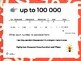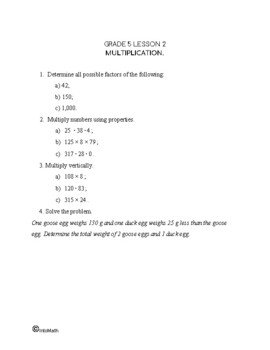CA\$2.00

100,000 (one hundred thousand) is the natural number following 99,999 and preceding 100,001.

In this math lesson you will learn about numbers up to 100,000 and how to do operations with these numbers.

You will discover their properties (for example, that any number added to 0 is that number). In addition, you will learn about the importance of the place value of each digit within the number.

Moreover, you will also learn how to pronounce and write these numbers in words correctly.

In this lesson, we demonstrate how to add and subtract numbers up to 100,000, as well as how to multiply and divide them vertically. The process involves  “borrowing” a digit or “carrying” a digit.

Our place-value system is called the “decimal” system, because it’s based on 10 fingers and when we move to the next ten, we add another zero, thus creating a new place value for a new digit.